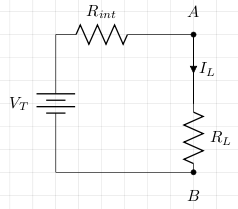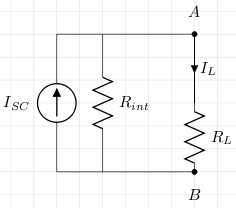# Equivalence Of Thevenin's And Norton's Theorems

According to Thevenin's theorem a two terminal power supply can be represented like this:And According to Norton's theorem the same power supply can be represented like this:From the Thevenin circuit we have

```IL = VT/(Rint+RL)
```

From the Norton circuit we have

```VAB = ISC(RintRL/(Rint+RL))
= ILRL
ILRL = ISC(RintRL/(Rint+RL))
IL = VAB/RL
= ISCRint/(Rint+RL)
```

VAB is the same for both circuits, so we can substitute what we stated IL was for the Thevenin circuit:

```ISCRint/(Rint+RL) = VT/(Rint+RL)
ISCRint = VT
```

Both theorems give us a way to represent complicated circuits as simpler ones, either as a simple series circuit, or a simple parallel circuit.

## References

Fischer-Cripps. A.C., The Electronics Companion. Institute of Physics, 2005.